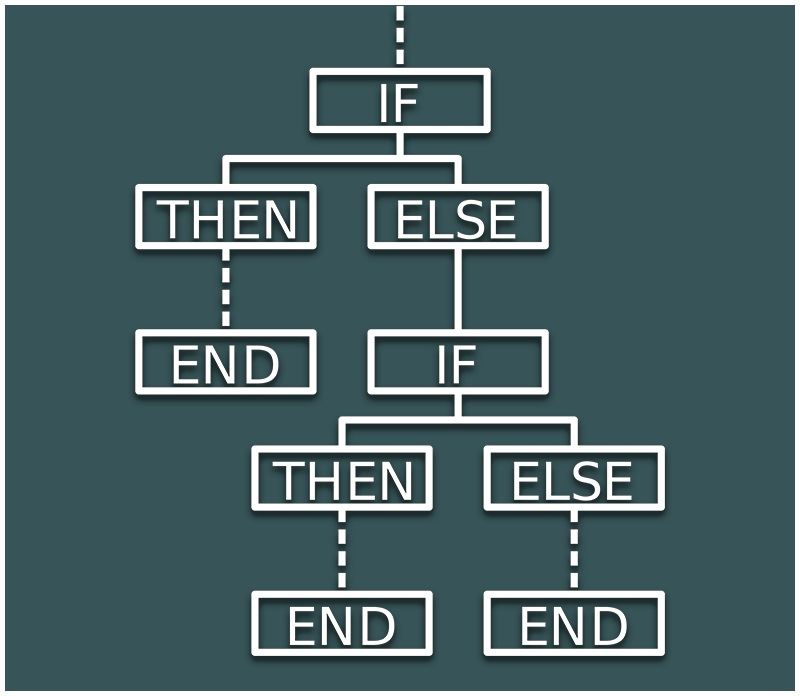Jet Set Go! All about Aeroplanes Jet Set Go! All about Aeroplanes

# Use of If and Then Statement

In mathematical reasoning, to infer a conclusion we frequently make use of if-then statements as:

P: If a and b are positive integers then their product is also positive.This sentence can be expressed as a component of two sentences.

a: a and b are positive integers

b: product of a and b is positive

The given statement implies that the product of both the numbers is positive if it is pre-mentioned that both the numbers are positive integers.

There are certain facts we can conclude from the given implication

(i) If a is false then we cannot say anything about the truth of b

(ii) The sentence does not imply that a surely happens.

(iii) If a is true then the only b is true.

## Contrapositive statement

To make a contrapositive statement from a given conditional (if-else statement), negate both the statements and then interchange the position of the statements them. Consider the following statement:

P: If two lines are parallel then they will have no common point of intersection.

The component statements would be:

a: Two lines are parallel

b: They will have no common point of intersection

For making the contrapositive of this statement, we negate both the statements as following;

~a= Two lines are parallel

~b=They will have a common point of intersection

Now the contrapositive statement will be:

S: If two lines have the common point of intersection then they are not parallel.

The meaning delivered by the statement P is same as S.

## Converse Statement

To write the converse of a statement, both the component statements are interchanged with each other.

Consider the following statement:

P: If any natural number n is divisible by 2 then n is even.

The component statements would be:

a:n is any natural number divisible by 2

b:n is even.

To write the converse we interchange both the statements as follows:

a: n is any even natural number

b:n is divisible by 2

The converse statement is given as:

S: If n is any even natural number then n is divisible by 2.

The meaning remains the same in case we take the converse of a conditional statement.

### Example

Let us take an example and try to find the contrapositive and converse of it.

Example: P: If two angles in a triangle are equal then the triangle is isosceles.

Solution: The contrapositive statement is given by:

S: A triangle is not isosceles if any two angles of a triangle are not equal

The converse statement would be:

S: If a triangle is isosceles then two angles of the triangle are equal

We have seen how to use contrapositive statements and converse statements in mathematical reasoning. To learn more about mathematical reasoning, please visit us at BYJU’S.

Test your knowledge on Use Of If Then Statements In Mathematical Reasoning